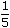# Aptitude - Partnership

### Exercise :: Partnership - General Questions

6.

A starts business with Rs. 3500 and after 5 months, B joins with A as his partner. After a year, the profit is divided in the ratio 2 : 3. What is B's contribution in the capital?

 A. Rs. 7500 B. Rs. 8000 C. Rs. 8500 D. Rs. 9000

Explanation:

Let B's capital be Rs. x.

 Then,3500 x 12 = 27x 314x = 126000x = 9000.

7.

A and B entered into partnership with capitals in the ratio 4 : 5. After 3 months, A withdrewof his capital and B withdrewof his capital. The gain at the end of 10 months was Rs. 760. A's share in this profit is:

 A. Rs. 330 B. Rs. 360 C. Rs. 380 D. Rs. 430

Explanation:

 A : B =4x x 3 +4x - 1 x 4xx 7:5x x 3 +5x - 1 x 5xx 74 5

= (12x + 21x) : (15x + 28x)

= 33x :43x

= 33 : 43.A's share = Rs.760 x 33= Rs. 330. 76

8.

A and B started a partnership business investing some amount in the ratio of 3 : 5. C joined then after six months with an amount equal to that of B. In what proportion should the profit at the end of one year be distributed among A, B and C?

 A. 3 : 5 : 2 B. 3 : 5 : 5 C. 6 : 10 : 5 D. Data inadequate

Explanation:

Let the initial investments of A and B be 3x and 5x.

A : B : C = (3x x 12) : (5x x 12) : (5x x 6) = 36 : 60 : 30 = 6 : 10 : 5.

9.

A, B, C rent a pasture. A puts 10 oxen for 7 months, B puts 12 oxen for 5 months and C puts 15 oxen for 3 months for grazing. If the rent of the pasture is Rs. 175, how much must C pay as his share of rent?

 A. Rs. 45 B. Rs. 50 C. Rs. 55 D. Rs. 60

Explanation:

A : B : C = (10 x 7) : (12 x 5) : (15 x 3) = 70 : 60 : 45 = 14 : 12 : 9.C's rent = Rs.175 x 9= Rs. 45. 35

10.

A and B started a business in partnership investing Rs. 20,000 and Rs. 15,000 respectively. After six months, C joined them with Rs. 20,000. What will be B's share in total profit of Rs. 25,000 earned at the end of 2 years from the starting of the business?

 A. Rs. 7500 B. Rs. 9000 C. Rs. 9500 D. Rs. 10,000

Explanation:

A : B : C = (20,000 x 24) : (15,000 x 24) : (20,000 x 18) = 4 : 3 : 3.B's share = Rs.25000 x 3= Rs. 7,500. 10